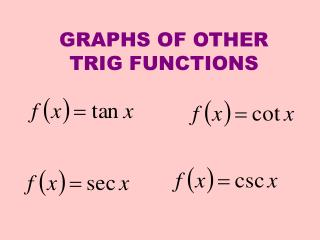Download PresentationGRAPHS OF OTHER TRIG FUNCTIONS

GRAPHS OF OTHER TRIG FUNCTIONS - PowerPoint PPT Presentation

GRAPHS OF OTHER TRIG FUNCTIONS. We are interested in the graph of y = f ( x ) = tan x. Start with a "t" chart and let's choose values from our unit circle and find the tangent values. Tangent has a period of  so it will repeat every . y. would mean there is a vertical asymptote here.I am the owner, or an agent authorized to act on behalf of the owner, of the copyrighted work described.
Download PresentationGRAPHS OF OTHER TRIG FUNCTIONS

Download Policy: Content on the Website is provided to you AS IS for your information and personal use and may not be sold / licensed / shared on other websites without getting consent from its author.While downloading, if for some reason you are not able to download a presentation, the publisher may have deleted the file from their server.

- - - - - - - - - - - - - - - - - - - - - - - - - - E N D - - - - - - - - - - - - - - - - - - - - - - - - - -
Presentation Transcript
1. GRAPHS OF OTHER TRIG FUNCTIONS

2. We are interested in the graph of y = f(x) = tan x Start with a "t" chart and let's choose values from our unit circle and find the tangent values. Tangent has a period of  so it will repeat every . y would mean there is a vertical asymptote here x y = tan x x

3. Since we went from we have one complete period Let's choose more values. y = tan x x y = tan x y x would mean there is a vertical asymptote here

4. Let's see what the graph would look like for y = tan x for 3 complete periods. The vertical lines are not part of the graph but are the asymptotes. If you use your graphing calculator it will probably put those in as well as showing the graph.

5. Transformations apply as usual. Let’s try one. up 2 reflect over x-axis right /4

6. Since the period of tangent is , the period of tan x is: The period would be /2 y = tan x y = tan 2x

7. What about the graph of y = f(x) = cot x? This would be the reciprocal of tangent so let's take our tangent values and "flip" them over. y x tan x y = cot x x

8. Let's choose more values. y = cot x x tan x y = cot x y x We need to see more than one period to get a good picture of this.

9. Let's look at the tangent graph again to compare these. y = cot x y = tan x Again the vertical lines are not part of the graph but are the asymptotes. Notice vertical asymptotes of one are zeros of the other.

10. For the graph of y = f(x) = csc x we'll take the reciprocals of the sine values. x sin x y = csc x y 1 x - 1 When we graph these rather than plot points after we see this, we'll use the sine graph as a sketching aid and then get the cosecant graph.

11. choose more values y = f(x) = csc x x sin x y = csc x When the sine is 0 the cosecant will have an asymptote. y 1 x - 1 We'll use the sine graph as the sketching aid.

12. Let's look over a few periods at the graph of y = csc x Let's add in the graph of the sine function so you can see how if you graph it, you can then easily use it to graph the cosecant. Again the vertical lines are not part of the graph but are where the cosecant is undefined (which is where the sine was 0 although this graphing program seemed to draw them a little to the right. They should cross the x-axis where the sine is 0)

13. For the graph of y = f(x) = sec x we'll take the reciprocal of the cosine values. x cos x y = sec x y 1 x - 1

14. Choose more values. y = f(x) = sec x x cos x y = sec x y 1 x - 1 Again the cosine graph will help graph the secant graph.

15. Let's look over a few periods at the graph of y =sec x Let's add in the graph of the cosine function so you can see how if you graph it, you can then easily use it to graph the secant. Again the vertical lines are not part of the graph but are where the secant is undefined (which is where the cosine was 0)